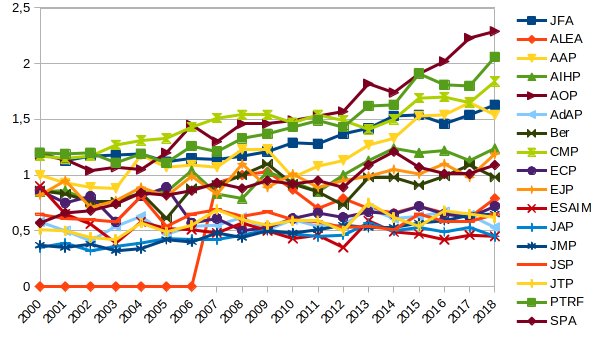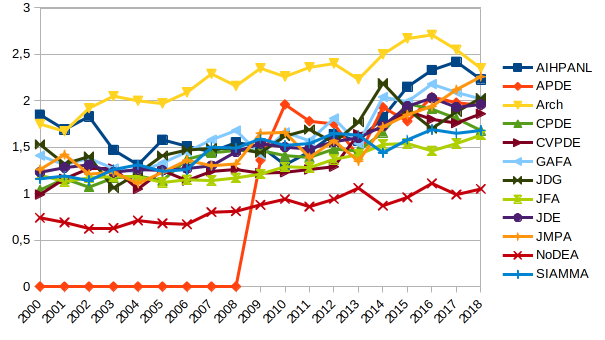# Category: UncategorizedActualité sur le front des publications mathématiques françaises :

This tiny post is devoted to the Hellinger distance and affinity.

Hellinger. Let ${\mu}$ and ${\nu}$ be probability measures with respective densities ${f}$ and ${g}$ with respect to the Lebesgue measure ${\lambda}$ on ${\mathbb{R}^d}$. Their Hellinger distance is

$\mathrm{H}(\mu,\nu) ={\Vert\sqrt{f}-\sqrt{g}\Vert}_{\mathrm{L}^2(\lambda)} =\Bigr(\int(\sqrt{f}-\sqrt{g})^2\mathrm{d}\lambda\Bigr)^{1/2}.$

This is well defined since ${\sqrt{f}}$ and ${\sqrt{g}}$ belong to ${\mathrm{L}^2(\lambda)}$. The Hellinger affinity is

$\mathrm{A}(\mu,\nu) =\int\sqrt{fg}\mathrm{d}\lambda, \quad \mathrm{H}(\mu,\nu)^2 =2-2A(\mu,\nu).$

This gives ${H(\mu,\nu)^2\in[0,2]}$, ${A(\mu,\nu)\in[0,1]}$, and the tensor product formula

$\mathrm{H}(\mu^{\otimes n},\nu^{\otimes n})^2 =2-2A(\mu^{\otimes n},\nu^{\otimes n}) =2-2A(\mu,\nu)^n =2-2\left(1-\frac{\mathrm{H}(\mu,\nu)^2}{2}\right)^n.$

Note that ${\mathrm{H}(\mu,\nu)^2=2}$ iff ${\mu}$ and ${\nu}$ have disjoint supports.

Note that if ${\mu\neq\nu}$ then ${\lim_{n\rightarrow\infty}\mathrm{H}(\mu^{\otimes n},\nu^{\otimes n})=2}$, a high dimensional phenomenon.

The notions of Hellinger distance and affinity pass to discrete distributions by replacing the Lebesgue measure ${\lambda}$ by the counting measure. The Hellinger distance above is a special case of the ${\mathrm{L}^p}$ version ${\Vert f^{1/p}-g^{1/p}\Vert_{\mathrm{L}^p(\lambda)}}$ available for arbitrary ${p\geq1}$. This is useful in asymptotic statistics, and we refer to the textbooks listed below.

Relation to total variation distance. The Hellinger distance is equivalent topologically and close metrically to the total variation distance, in the sense that

$\mathrm{H}^2(\mu,\nu) \leq\left\Vert\mu-\nu\right\Vert_{\mathrm{TV}} \leq\mathrm{H}(\mu,\nu)\sqrt{4-\mathrm{H}(\mu,\nu)^2} \leq2\mathrm{H}(\mu,\nu)$

where

$\left\Vert\mu-\nu\right\Vert_{\mathrm{TV}} =\sup_A|\mu(A)-\nu(A)| =\int|f-g|\mathrm{d}\lambda.$

Indeed, the first inequality comes from the following elementary observation

$(\sqrt{a}-\sqrt{b})^2 =a+b-2\sqrt{ab} \leq a+b-2(a\wedge b) =|a-b|,$

valid for all ${a,b\geq0}$, while the second inequality comes from

$|a-b|=|\sqrt{a}^2-\sqrt{b}^2|=|\sqrt{a}-\sqrt{b}|(\sqrt{a}+\sqrt{b})$

whiche gives, thanks to the Cauchy-Schwarz inequality,

$\int|f-g|\mathrm{d}\lambda \leq\mathrm{H}(\mu,\nu)\sqrt{\int(\sqrt{f}+\sqrt{g})^2\mathrm{d}\lambda} =\mathrm{H}(\mu,\nu)\sqrt{2+2A(\mu,\nu)}.$

Gaussian explicit formula. The Hellinger distance (or affinity) between two Gaussian distributions can be computed explicitly, just like the square Wasserstein distance and the Kullback-Leibler divergence or relative entropy. Namely

$\mathrm{A}(\mathcal{N}(m_1,\sigma_1^2),\mathcal{N}(m_2,\sigma_2^2)) =\sqrt{2\frac{\sigma_1\sigma_2}{\sigma_1^2+\sigma_2^2}} \exp\Bigr(-\frac{(m_1-m_2)^2}{4(\sigma_1^2+\sigma_2^2)}\Bigr),$

equal to ${1}$ iff ${(m_1,\sigma_1)=(m_2,\sigma_2)}$. By using the tensor product formula, we have also

$\mathrm{A}(\mathcal{N}(m_1,\sigma_1^2)^n,\mathcal{N}(m_2,\sigma_2^2)^n) =\Bigr(2\frac{\sigma_1\sigma_2}{\sigma_1^2+\sigma_2^2}\Bigr)^{n/2} \exp\Bigr(-n\frac{(m_1-m_2)^2}{4(\sigma_1^2+\sigma_2^2)}\Bigr).$

Here is a general “matrix” formula for Gaussians on ${\mathbb{R}^d}$, ${d\geq1}$, with ${\Delta m=m_2-m_1}$,

$\mathrm{A}(\mathcal{N}(m_1,\Sigma_1),\mathcal{N}(m_2,\Sigma_2)) =\frac{\det(\Sigma_1\Sigma_2)^{1/4}}{\det(\frac{\Sigma_1+\Sigma_2}{2})^{1/2}} \exp\Bigr(-\frac{\langle\Delta m,(\Sigma_1+\Sigma_2)^{-1}\Delta m)\rangle}{4}\Bigr).$

The Hellinger affinity is also known as the Bhattacharyya coefficient, and enters the definition of the Bhattacharyya distance ${(\mu,\nu)\mapsto-\log\mathrm{A}(\mu,\nu)}$.

Application to long time behavior of Ornstein-Uhlenbeck. Let ${{(B_t)}_{t\geq0}}$ be an ${n}$-dimensional standard Brownian motion and let ${{(X^x_t)}_{t\geq0}}$ be the Ornstein-Uhlenbeck process solution of the stochastic differential equation

$X_0=x,\quad \mathrm{d}X^x_t=\sqrt{2}\mathrm{d}B_t-X^x_t\mathrm{d}t$

where ${x\in\mathbb{R}^n}$. By plugging this equation into the identity ${\mathrm{d}(\mathrm{e}^tX^x_t)=\mathrm{e}^t\mathrm{d}X^x_t+\mathrm{e}^tX^x_t\mathrm{d}t}$ we get the Mehler formula (the variance comes from the Wiener integral)

$X^x_t=x\mathrm{e}^{-t}+\sqrt{2}\int_0^t\mathrm{e}^{s-t}\mathrm{d}B_s \sim \mathcal{N}(x\mathrm{e}^{-t},(1-\mathrm{e}^{-2t})I_n) \underset{t\rightarrow\infty}{\longrightarrow} \mathcal{N}(0,I_n).$

It follows in particular that for all ${x,y\in\mathbb{R}^n}$ an ${t>0}$

$\frac{1}{2}\mathrm{H}^2(\mathrm{Law}(X^x_t),\mathrm{Law}(X^y_t)) =1-\exp\Bigr(-\frac{|x-y|^2\mathrm{e}^{-2t}}{1-\mathrm{e}^{-2t}}\Bigr).$

Moreover, denoting ${\mu_t=\mathrm{Law}(X^x_t)}$ and ${\mu_\infty=\mathcal{N}(0,I_n)}$, it follows that

$\mathrm{H}(\mu_t,\mu_\infty)^2 =2-2\Bigr(2\frac{\sqrt{1-\mathrm{e}^{-2t}}}{2-\mathrm{e}^{-2t}}\Bigr)^{1/2} \exp\Bigr(-\frac{|x|^2\mathrm{e}^{-2t}}{4(2-\mathrm{e}^{-2t})}\Bigr).$

This quantity tends to ${0}$ as ${t\rightarrow\infty}$. If ${|x|^2=x_1^2+\cdots+x_n^2\sim cn}$ then this happens, as ${n}$ is large, near the critical value ${t=\frac{1}{2}\log(n)}$, for which ${\mathrm{e}^{-2t}=1/n}$. More information about cutoffs phenomena for Ornstein-Uhlenbeck and diffusions is available in the papers below.

This post gives below the Mathematical Citation Quotient (MCQ) from 2000 to 2018 for journals in probability, statistics, analysis, and general mathematics. The numbers were obtained using home brewed scripts and MathSciNet data. The graphics were created with LibreOffice.

Recall that the MCQ is a ratio of two counts for a selected journal and a selected year.  The MCQ for year $Y$ and journal $J$ is given by the formula $\mathrm{MCQ}=m/n$ where

• $m$ is the total number of citations of papers published in jounal $J$ in years $Y-1$,…,$Y-5$ by papers published in year $Y$ in any journal known by MathSciNet;
• $n$ is the total number of papers published in journal $J$ in years $Y-1$,…,$Y-5$.

The Mathematical Reviews compute every year the MCQ for every indexed journal, and make it available on MathSciNet. This formula is very similar to the one of the five years impact factor, the main difference being the population of journals which is specifically mathematical for the MCQ (reference list journals) and the way the citations are extracted. Both biases are negative.

The MCQ is a rough measurement of the social scientific value of journals. The results are quite compatible with what we have in mind. The trends are sometimes intriguing. For probability journals, for instance, it seems that there are three groups. This reminds reinforcement or self-organized criticality. The first group is AOP-PTRF-CMP-JFA-AAP, with some hesitations and an “Annals” naming effect. The second group is AIHP-EJP-SPA-Bernoulli, the third group is ALEA-ECP-AdAP-JAP-JTP-ESAIM. We observe some transitions from one group to another, for instance, since 2010, AAP moved to the first group while ALEA moved to the third group. The case of ECP is very special since its papers are half the standard size. The MCQ probably underestimates the social value of ECP by a rough factor 2, which is logical if we compare with EJP.

Yes, there are more robust ways to measure the social value of a journal, such as for instance the (recursive) eigenfactor, and it could be interesting to check if the three groups are stable!Σ

Let $X=(X_1,\ldots,X_n)$ be a random vector of $(\mathbb{R}^d)^n$ with density proportional to $$(x_1,\ldots,x_n)\in(\mathbb{R}^d)^n\mapsto\mathrm{e}^{-\beta\sum_{i=1}^nV(x_i)}\prod_{i<j}W(x_i-x_j),$$ where $V,W:\mathbb{R}^d\to\mathbb{R}$ are homogeneous functions, with $W\geq0$. This means that there exist $a,b\geq0$ such that for all $\lambda\geq0$ and $x\in\mathbb{R}^d$, $V(\lambda x)=\lambda^a V(x)$ and $W(\lambda x)=\lambda^bW(x)$. Now, for all $\theta>0$, by the change of variable $x_i=\sqrt[a]{\beta/(\theta+\beta)}y_i$,
\begin{multline*}
\int_{(\mathbb{R}^d)^n}\mathrm{e}^{-(\theta+\beta)\sum_iV(x_i)}\prod_{i<j}W(x_i-x_j)\mathrm{d}x\\
=\Bigr(\frac{\beta}{\theta+\beta}\Bigr)^{\frac{nd}{a}+\frac{n(n-1)a}{2b}}
\int_{(\mathbb{R}^d)^n}\mathrm{e}^{-\beta\sum_iV(y_i)}\prod_{i<j}W(y_i-y_j)\mathrm{d}y.
\end{multline*}
We recognize the Laplace transform of a Gamma distribution, since
$\int_0^\infty\mathrm{e}^{-\theta u}u^{\alpha-1}\mathrm{e}^{-\beta u}\mathrm{d}u =\int_0^\infty u^{\alpha-1}\mathrm{e}^{-(\theta+\beta)u}\mathrm{d}u =\Bigr(\frac{\beta}{\theta+\beta}\Bigr)^\alpha\frac{\Gamma(\alpha)}{\beta^\alpha},$and we obtain
$\sum_iV(X_i)\sim\mathrm{Gamma}\Bigr(\frac{nd}{a}+\frac{n(n-1)bd}{2a},\beta\Bigr).$
A remarkable general fact! The case $V=\frac{1}{2}\left|\cdot\right|^2$ and $W=\left|\cdot\right|^\beta$ corresponds to the beta Ginibre gas of random matrix theory. The case $V=\frac{n+1}{2}\log(1+\left|\cdot\right|^2)$ and $W=\left|\cdot\right|^2$ corresponds to the Forrester–Krishnapur spherical gas of random matrix theory.

We could generalize even more,  and replace $(x_1,\ldots,x_n)\mapsto\sum_iV(x_i)$ by a homogenenous $(x_1,\ldots,x_n)\mapsto V(x_1,\ldots,x_n)$ and $(x_1,\ldots,x_n)\mapsto\prod_{i<j}W(x_i-x_j)$ by a homogeneous $(x_1,\ldots,x_n)\mapsto W(x_1,\ldots,x_n)$, in the sense that for some $a,b\geq0$ and all $\lambda\geq0$, $x\in(\mathbb{R}^d)^n$, $V(\lambda x)=\lambda^aV(x)$ and $W(\lambda x)=\lambda^bW(x)$. In this case $X=(X_1,\ldots,X_n)$ has density proportional to $x\in(\mathbb{R}^d)^n\mapsto\mathrm{e}^{-\beta V(x)}W(x)$. This would hide the structure of exchangeable gas with pair-interaction that we had in mind for the examples. But this would give $$V(X)=V(X_1,\ldots,X_n)\sim\mathrm{Gamma}\Bigr((n+b)\frac{d}{a},\beta\Bigr).$$

Syntax · Style · Tracking & Privacy.